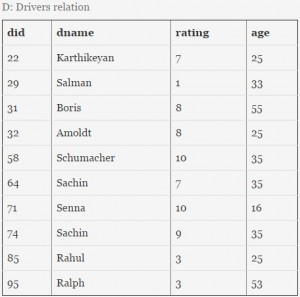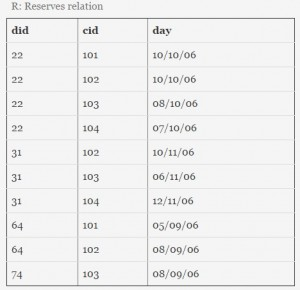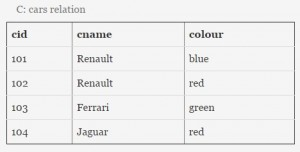# GATE | GATE IT 2006 | Question 86

Consider a database with three relation instances shown below. The primary keys for the Drivers and Cars relation are did and cid respectively and the records are stored in ascending order of these primary keys as given in the tables. No indexing is available in the database.Let n be the number of comparisons performed when the above SQL query is optimally executed. If linear search is used to locate a tuple in a relation using primary key, then n lies in the range
(A) 36 – 40
(B) 44 – 48
(C) 60 – 64
(D) 100 – 104

Explanation:

here we have to calculate the number of comparisons performed when the above SQL query is optimally executed.

from the first inner query:

select R.did from Cars C, Reserves R
where R.cid = C.cid and C.colour = ‘red’

C.color = “Red”, comparisons=4 (Cars has four rows)

R.cid=C.cid so there are five rows extracted to this where condition.
comparisons=(2 red cars * 10 Reserves rows)=20

from the second inner query:
select R.did from Cars C, Reserves R
where R.cid = C.cid and C.colour = ‘green’

C.color = “Green”, comparisons=4 (Cars has four rows)

R.cid=C.cid so there are three rows extracted to this where condition.
comparisons=(1 green car*10 Reserves rows)=10

R.did = {22, 22, 31,31, 64} for first inner query
R.did = {22, 31, 74} for second inner query

Here unique sets are, R.did={22,31,64} and R.did={22,31,74} respectively for first and second inner queries.
so for intersection, 6 comparisons (for 22, we hit on the first try and for 31,we hit on the second try,and for 74,we hit on all three try, so comaprisons=1+2+3)
Finally we have to locate the did – 22 and did 31 from the driver table and did is the primary key. As told in the question, we use linear search and for 22,
we hit on the first try and for 31 we hit on the third try. So, 1 + 3 = 4 comparisons.
so total no of comparisons= 4+20+4+10+6+4=48

In short: So, first get 2 red cars by scanning 4 tuples of the cars relation. Now, for each of the two ‘red’ cars, we scan all the 10 tuples of the ‘Reserves’ relation and thus we get 2*10 + 4 = 24 comparisons.Similarly for the ‘green’ car we get 4+10 = 14 comparisons.

This solution is contributed by Nitika Bansal.

Quiz of this Question

My Personal Notes arrow_drop_up
Article Tags :

3

Please write to us at contribute@geeksforgeeks.org to report any issue with the above content.